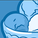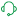# New to Qlik Sense

If you’re new to Qlik Sense, start with this Discussion Board and get up-to-speed quickly.

Announcements
QlikWorld starts MONDAY! last chance to register is now ! REGISTER NOW
cancel
Showing results for
Did you mean:Contributor III

## Highlight Consecutive Values Control Chart

Hi All 🙂

I have a process control chart and would like to highlight the data points say for example if 6 points are consecutively on one side of the mean however I am unsure how to write this.

Please see pic to see what I would like to highlight, many thanks! 😄Labels (4)

• ### SaaS

1 Solution

Accepted SolutionsSpecialist

This is not pretty, but it should work.  You would use the Above function to check previous and following points.  Set as Color as Expression.  This is the start.  You would need to finish the combinations where I have . . . and replace Value and SDate with you measure and dimension.

if((sum(Value)>(sum(Total Value)/count(Distinct Total SDate)) and
above(sum(Value),1)>(sum(Total Value)/count(Distinct Total SDate)) and
above(sum(Value),2)>(sum(Total Value)/count(Distinct Total SDate)) and
above(sum(Value),3)>(sum(Total Value)/count(Distinct Total SDate)) and
above(sum(Value),4)>(sum(Total Value)/count(Distinct Total SDate)) and
above(sum(Value),5)>(sum(Total Value)/count(Distinct Total SDate))) or
(sum(Value)>(sum(Total Value)/count(Distinct Total SDate)) and
above(sum(Value),-1)>(sum(Total Value)/count(Distinct Total SDate)) and
above(sum(Value),1)>(sum(Total Value)/count(Distinct Total SDate)) and
above(sum(Value),2)>(sum(Total Value)/count(Distinct Total SDate)) and
above(sum(Value),3)>(sum(Total Value)/count(Distinct Total SDate)) and
above(sum(Value),4)>(sum(Total Value)/count(Distinct Total SDate))) or
(sum(Value)>(sum(Total Value)/count(Distinct Total SDate)) and
above(sum(Value),-2)>(sum(Total Value)/count(Distinct Total SDate)) and
above(sum(Value),-1)>(sum(Total Value)/count(Distinct Total SDate)) and
above(sum(Value),1)>(sum(Total Value)/count(Distinct Total SDate)) and
above(sum(Value),2)>(sum(Total Value)/count(Distinct Total SDate)) and
above(sum(Value),3)>(sum(Total Value)/count(Distinct Total SDate))) or

. . . .

,Red(),Black())

3 RepliesSupport (Former)

I believe that is likely going to need Set Analysis, but I am not sure, the following may help if that is the case:

https://community.qlik.com/t5/Qlik-Design-Blog/A-Primer-on-Set-Analysis/ba-p/1468344

https://community.qlik.com/t5/Qlik-Design-Blog/Set-Analysis-in-the-Aggr-function/ba-p/1463822

Here is the Help link to it too:

https://help.qlik.com/en-US/sense/November2020/Subsystems/Hub/Content/Sense_Hub/ChartFunctions/SetAn...

Sorry I am not more help, I am not very good at the dev side of things, more of a backend guy, apologies.

Regards,
Brett

To help users find verified answers, please do not forget to use the "Accept as Solution" button on any post(s) that helped you resolve your problem or question.
I now work a compressed schedule, Tuesday, Wednesday and Thursday, so those will be the days I will reply to any follow-up posts.Specialist

This is not pretty, but it should work.  You would use the Above function to check previous and following points.  Set as Color as Expression.  This is the start.  You would need to finish the combinations where I have . . . and replace Value and SDate with you measure and dimension.

if((sum(Value)>(sum(Total Value)/count(Distinct Total SDate)) and
above(sum(Value),1)>(sum(Total Value)/count(Distinct Total SDate)) and
above(sum(Value),2)>(sum(Total Value)/count(Distinct Total SDate)) and
above(sum(Value),3)>(sum(Total Value)/count(Distinct Total SDate)) and
above(sum(Value),4)>(sum(Total Value)/count(Distinct Total SDate)) and
above(sum(Value),5)>(sum(Total Value)/count(Distinct Total SDate))) or
(sum(Value)>(sum(Total Value)/count(Distinct Total SDate)) and
above(sum(Value),-1)>(sum(Total Value)/count(Distinct Total SDate)) and
above(sum(Value),1)>(sum(Total Value)/count(Distinct Total SDate)) and
above(sum(Value),2)>(sum(Total Value)/count(Distinct Total SDate)) and
above(sum(Value),3)>(sum(Total Value)/count(Distinct Total SDate)) and
above(sum(Value),4)>(sum(Total Value)/count(Distinct Total SDate))) or
(sum(Value)>(sum(Total Value)/count(Distinct Total SDate)) and
above(sum(Value),-2)>(sum(Total Value)/count(Distinct Total SDate)) and
above(sum(Value),-1)>(sum(Total Value)/count(Distinct Total SDate)) and
above(sum(Value),1)>(sum(Total Value)/count(Distinct Total SDate)) and
above(sum(Value),2)>(sum(Total Value)/count(Distinct Total SDate)) and
above(sum(Value),3)>(sum(Total Value)/count(Distinct Total SDate))) or

. . . .

,Red(),Black())Specialist

This isn't pretty, but it should highlight the correct values.  In Color by Expression, this uses the Above function to check previous and following values.  This is just the start, you will need to finish the pattern for possible combinations where I have . . . and replace Value with your measure and SDate with you Dimension.

if((sum(Value)>(sum(Total Value)/count(Distinct Total SDate)) and
above(sum(Value),1)>(sum(Total Value)/count(Distinct Total SDate)) and
above(sum(Value),2)>(sum(Total Value)/count(Distinct Total SDate)) and
above(sum(Value),3)>(sum(Total Value)/count(Distinct Total SDate)) and
above(sum(Value),4)>(sum(Total Value)/count(Distinct Total SDate)) and
above(sum(Value),5)>(sum(Total Value)/count(Distinct Total SDate))) or
(sum(Value)>(sum(Total Value)/count(Distinct Total SDate)) and
above(sum(Value),-1)>(sum(Total Value)/count(Distinct Total SDate)) and
above(sum(Value),1)>(sum(Total Value)/count(Distinct Total SDate)) and
above(sum(Value),2)>(sum(Total Value)/count(Distinct Total SDate)) and
above(sum(Value),3)>(sum(Total Value)/count(Distinct Total SDate)) and
above(sum(Value),4)>(sum(Total Value)/count(Distinct Total SDate))) or
(sum(Value)>(sum(Total Value)/count(Distinct Total SDate)) and
above(sum(Value),-2)>(sum(Total Value)/count(Distinct Total SDate)) and
above(sum(Value),-1)>(sum(Total Value)/count(Distinct Total SDate)) and
above(sum(Value),1)>(sum(Total Value)/count(Distinct Total SDate)) and
above(sum(Value),2)>(sum(Total Value)/count(Distinct Total SDate)) and
above(sum(Value),3)>(sum(Total Value)/count(Distinct Total SDate))) or

. . . .

,Red(),Black())